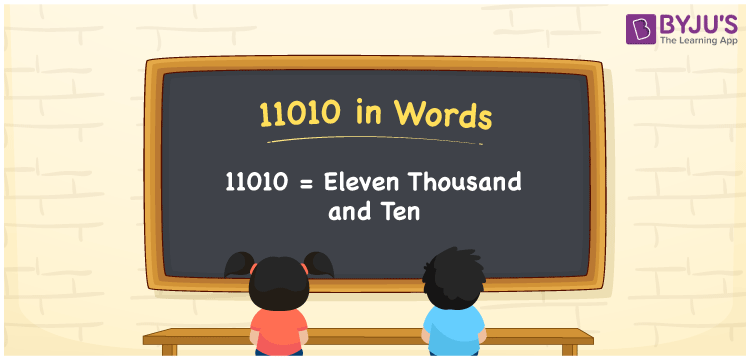# 11010 in Words

We can write 11010 in words as Eleven thousand and ten. If you bought a portable fan that cost Rs. 11010, then you can say, “I bought a portable fan worth Eleven thousand and ten rupees”. Using a place value chart, we can easily convert the cardinal number 11010 into words. In this article, you will understand how to write 11010 in words and some interesting facts about 11010.

 11010 in words Eleven thousand and ten Eleven thousand and ten in Numbers 11010

## 11010 in English Words

Generally, we write numbers in words using the English alphabet. Thus, we spell 11010 in English words as “Eleven thousand and ten”.## How to Write 11010 in Words?

The number 11010 contains five digits. Let us make a place value chart with columns, as shown below.

 Ten thousands Thousands Hundreds Tens Ones 1 1 0 1 0

Here, ones = 0, tens = 1, hundreds = 0, thousands = 1, ten thousands = 1

So, 1 × Ten thousand + 1 × Thousand + 0 × Hundred + 1 × Ten + 0 × One

= 1 × 10000 + 1 × 1000 + 0 × 100 + 1 × 10 + 0 × 1

= 10000 + 1000 + 10

= Ten Thousand + One thousand + Ten

= Eleven thousand + Ten

= Eleven thousand and ten

11010 is a natural number that is the successor of 11009 and predecessor of 11011.

11010 in words – Eleven thousand and ten

Is 11010 an odd number? – No

Is 11010 an even number? – Yes

Is 11010 a prime number? – No

Is 11010 a composite number? – Yes

Is 11010 a perfect square number? – No

Is 11010 a perfect cube number? – No

## Frequently Asked Questions on 11010 in Words

Q1

### How to write 11010 in words?

The number 11010 can be written in words as Eleven thousand and ten.
Q2

### How do you write Rs. 11010 in words on a cheque?

On a cheque, we can express an amount of Rs. 11010 in words as “Eleven thousand and ten rupees only”.
Q3

### Find the value of 11010 + 490.

11010 + 490 = 11500 Therefore, the value of 11010 + 490 is 11500.